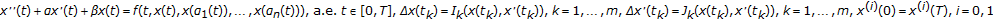# Existence of Solutions of Periodic Boundary Value Problems for Impulsive Functional Duffing Equations at Nonresonance Case

## Abstract

This paper deals with the existence of solutions of the periodic boundary value problem of the impulsive Duffing equations:. Sufficient conditions are established for the existence of at least one solution of above-mentioned boundary value problem. Our method is based upon Schaeffer's fixed-point theorem. Examples are presented to illustrate the efficiency of the obtained results.

## Author information

Authors

### Corresponding author

Correspondence to Xingyuan Liu.

## Rights and permissions

Reprints and Permissions

Liu, X., Liu, Y. Existence of Solutions of Periodic Boundary Value Problems for Impulsive Functional Duffing Equations at Nonresonance Case. Bound Value Probl 2008, 937138 (2008). https://doi.org/10.1155/2008/937138

• Accepted:

• Published:

• DOI: https://doi.org/10.1155/2008/937138

### Keywords

• Differential Equation
• Partial Differential Equation
• Ordinary Differential Equation
• Functional Equation
• Periodic Boundary